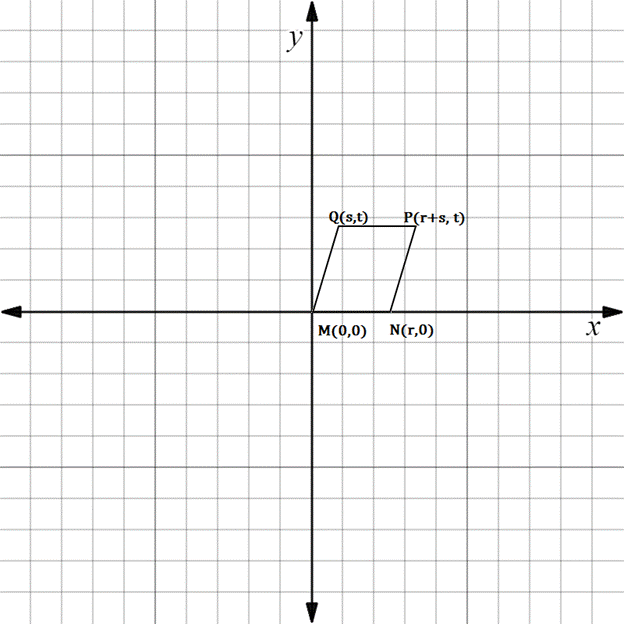Chapter 10.3, Problem 23E### Elementary Geometry for College St...

6th Edition
Daniel C. Alexander + 1 other
ISBN: 9781285195698

#### Solutions

Chapter
Section### Elementary Geometry for College St...

6th Edition
Daniel C. Alexander + 1 other
ISBN: 9781285195698
Textbook Problem
1 views

# In Exercises 23 to 28, find the equation (realtionship) requested. Then eliminate fractions and square root radicals from the equation.If ▱ M N P Q is a rhombus, state an equation that relates r , s , and t .To determine

To state:

An equation that relates r,s, and t for the given rhombus.

Explanation

The given figure is shown below.

If the given figure represents a rhombus, it is necessary that MN=MQ.

First find the distance MN.

From the figure it is observed that the distance MN=r.

To find the distance MQ, we have to use the distance formula.

x1,y1=M0, 0

x2,y2=Qs, t

Formula for distance between two points

d=x2-x12+y2-y12

Substituting the x and y co-ordinates

dMQ=s-02+

### Still sussing out bartleby?

Check out a sample textbook solution.

See a sample solution

#### The Solution to Your Study Problems

Bartleby provides explanations to thousands of textbook problems written by our experts, many with advanced degrees!

Get Started

#### Find more solutions based on key concepts